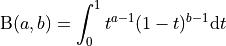# aesara.tensor.random.betabinom#

aesara.tensor.random.betabinom = <aesara.tensor.random.basic.BetaBinomialRV object>[source]#

A beta-binomial discrete random variable.

The probability mass function for betabinom in terms of its shape parameters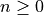,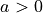,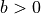and the probability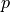is: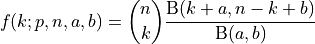where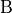is the beta function: